I have hear the words “Markov” more than a few times, but it is only recently that I can appreciate exactly what this simplification buys me. Markov was a guy who liked to model things by using only the current state. This simplification is often very appropriate and often offers a relatively accurate approximation depending on the problem at hand. It will at least be more accurate than not doing anything, and is considered a common computer science tool when modeling something.

For example:

### A Markov Chain for a “Black Friday Sale” ShopperIn the above example, the links represent the probability of a transition to a new state given the current state. The “Markov Property” is seen by how the probability of any transition is only dependent on the current state. It’s a pretty straightforward idea that I’ll leave to Wikipedia to better explain here.

## Example: Dealing with Temporal Uncertainty

We will explore the advantages of the “Markov Property” for simplifying the calculation of time-based processes. Below is an example showing how to tell if a Black Friday shopper is ready to check out. For this example, we only have one “evidence variable”. We can see whether or not the cart is full. The “Markov Assumption” that we will take is that the current state is only dependent on the previous state. The state of “cart is full” is represented by C, and the state of “Ready to leave” is represented by R. We will also assume that whether or not the cart is currently full is not dependent on information from the previous state (suspend disbelief).The graph above basically says “if their cart is full, they probably want to leave, otherwise, they probably don’t. If they wanted to leave last time we checked, they probably still want to leave, but maybe not. The nerd name for what we have drawn above is a “Temporal Bayesian Network”. We are also making the “Markov Assumption” that the current state is only affected by the state before this. If we did not do this, we would have to take all previous states into account when calculating each new state. Let’s do an example now!

Below is a table of evidence for a Black Friday shopper. We are going to calculate the probability that the shopper is ready to go at any given time.

Time Cart
6:00 Not Full
6:05 Full
6:10 Not Full
6:15 Full
6:20 Full

And that’s all the data we have. Let’s calculate the first time probability by hand.

For the first “ready to go” calculation, you have to make up a previous “steady state” probability about whether or not the shopper is ready to go. For this example, we’ll assume it’s .5. If any of these terms are weird, don’t worry, I’ll explain them afterwards.

Step 1: Calculate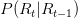$P(R_t | R_{t-1})$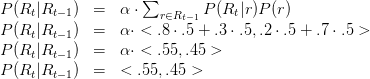$\begin{array}{lcl} P(R_t|R_{t-1}) & = & \alpha \cdot \sum_{r \in R_{t-1}}P(R_t|r)P(r) \\ P(R_t|R_{t-1}) & = & \alpha \cdot < .8 \cdot .5 + .3 \cdot .5 , .2 \cdot .5 + .7 \cdot .5 > \\ P(R_t|R_{t-1}) & = & \alpha \cdot < .55 , .45 > \\ P(R_t|R_{t-1}) & = & < .55 , .45 > \end{array}$

For this calculation, we need to sum up the different probabilities of$R_t$ given the different possibilities for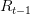$R_{t-1}$. Because each of those is affected by the likelihood of$R_{t-1}$, that probability is multiplied in. This is called the chain rule. The α character you see is there to re-weigh the true / false probabilities back to 1. This wasn’t necessary for that example, but it usually is.

Also, the step we have done above is called “filtering”. It is when we calculate the present state based on past information.

Now that we have accounted for the past in the current probability, it’s time to account for the current evidence available to us.

Step 2: Calculate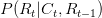$P(R_t | C_t, R_{t-1})$$\begin{array}{lcl} P(R_t| \neg C_t, R_{t-1}) & = & \alpha \cdot P(R_t|\neg C) \cdot P(R_t|R_{t-1})\\ & = & \alpha \cdot < .55 \cdot .4 , .45 \cdot .6 > \\ & = & \alpha \cdot < .22 , .27 > \\ & = & < .44898 , .55102 > \end{array}$

This was done assuming that the existence of a cart in time step t is independent from whether or not the person was ready to go in time step t – 1. As both these variables have been deemed independent, the probability of both of them happening is simply a multiplication.

Let’s go back and add another evidence variable that is dependent on the person’s state instead of the other way around. We will say that a person who wants to leave is probably holding a box of cigarettes. According to PBS, 20 percent of Americans smoke, so if we make the assumption that those people make it obvious they need to smoke by holding out cigarettes and that 25% of the time they just end up holding out a box of cigarettes for no apparent reason, that would create the following network (we will use B to represent a box of cigarettes being visible):

### A more interesting exampleAnd here’s an updated version of our first chart.

Time Cart Cigarettes Visible
6:00 Not Full No
6:05 Full No
6:10 Not Full No
6:15 Full Yes
6:20 Full No

Thanks to everything being independent from each other, we just need to add another equation onto the answer we already have:

Step 2: Calculate$P(R_t | B_t)$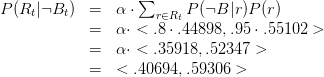$\begin{array}{lcl} P(R_t|\neg B_t) & = & \alpha \cdot \sum_{r \in R_t} P(\neg B|r)P(r) \\ & = & \alpha \cdot <.8 \cdot .44898, .95 \cdot .55102> \\ & = & \alpha \cdot < .35918 , .52347 > \\ & = & < .40694 , .59306 > \end{array}$

So far, it looks like this person is more likely to not be ready to go after one time step.

### Coding it up

For dealing with temporal networks, we can use the Hidden Markov Model to convert all of these calculations into matrices. Below is what I believe is a correct simulation of what is done above implemented in Octave (or Matlab).

% An Octave Example function Rt = markovNet ( Evidence ) Rt = [.5 .5]; % Initialize to some value Transition = [ .8 .2 ; .3 .7]; Cart = {[.4 0; 0 .6], [ .9 0; 0 .1]}; % {F, T} Cigarettes = {[.8 0; 0 .95], [ .2 0 ; 0 .05 ]}; % {F , T};   % go through each time step. for t = Evidence' Rt = Rt * Transition; Rt = Rt * Cart{t(1) + 1}; Rt = Rt * Cigarettes{t(2) + 1}; Rt = Rt ./ (Rt * ones(size(Rt))'); end end markovNet([ 0 0; 1 0; 0 0; 1 1; 1 0])

Assuming that i didn’t do something wrong in the code above, the probability that the person is ready to leave at 6:20 is 96.6%.

## Conclusion

If you ever wanted to figure out how to deal with a probability, using the Markov Assumption can help you considerably in making the problem more simple to model. It is not a 100% solution, but most of the time, it can get you close. Very close.

This site uses Akismet to reduce spam. Learn how your comment data is processed.